Subscribe to our newsletter to receive the latest news and events from TWI:

# Modelling Long Range Ultrasonic Waves in Complex Structures

## Modelling of Long Range Ultrasonic Waves in Complex Structures

Slim Soua, Septimonette Chan, Tat-Hean Gan

TWI Ltd, Granta Park, Great Abington, Cambridge CB21 6AL, UK

Paper presented at BINDT annual conference 2008, 15-18 September 2008, Macclesfield, Cheshire, UK.

## Abstract

At a fixed frequency dispersion curves give fundamental informations on guided waves (wavelength, dispersion, phase and group velocities and the travelling time of each mode ...). In long range ultrasonic NDT inspection, the use of modelling can be used to predict the best choice of experimental conditions.

There is commercial software that produces dispersion curves (DC), e.g. Disperse®, however this software only works for cylindrical and plate structures. The solution of Disperse® is based on analytical solution of wave equation in the frequency domain. The Fourier Transform is used to convert the time equations in frequency domain and all variables are harmonic in infinite time.

The purpose of this project is to develop a Semi Analytical Finite Element Method (SAFEM) to obtain phase and group velocity dispersion curves, in order to generate ultrasonic guided waves for bar like structures with complex cross section.

The advantages of the SAFEM are:

• Deal with complex cross section structure.
• Fast calculation time.
• Small computational memory required compared with the ordinary finite element method.

In addition to dispersion curves, this method can be used to carry out a complete modelling of the modal propagation in complex cross section structures.

This work provides a powerful numerical model in the Non Destructive Testing (NDT) understanding. The numerical platform is then used to make expertise and provide database for several complicated situations such as I-beam inspection.

## 1. Introduction

Dispersion curves evaluation has been the subject of many researches, among the older research Nigro in 1966 developed a semi-analytic solution based on function expansion of displacements called the Ritz method. Some difficulties were observed when dealing with convergence and the method was applied to square cross section bars. In the last 10 years analytical methods based on function expansion were not used because of their limitation to simple cross section and also because of their complexity. The finite element technique has been applied in all modelling fields in mechanics, especially in Long Range Ultrasonic (LRU) for the calculation of dispersion curves and mode characteristics. This method was also subjected to some limitation; mainly the memory used in such application is high because of the model length. SAFEM has been presented as an alternative as it avoids the 3D meshing and uses only 2D meshing. This is thanks to the analytical form of the considered displacements, the 3rd direction is simplified and 2D meshing is used for 3D displacement unknowns. In 1998 Volovoi affirms that until that date dispersion curves were studied almost exclusively for rectangular or circular cross sections, while dispersion information for other important sectional geometries remains very scarce. He presented the SAFEM used to generate dispersion curves for non-homogeneous anisotropic I-beams. Gavric used the SAFEM for LRU applications, he obtained the dispersion curves for propagative (real) and evanescent (complex) in a straight wave guide. The results are shown for a free rail for several deformations as axial, vertical flexion, horizontal flexion, torsional and other types of propagative waves which can not be described using analogies with simple beam waves. Gavric shows that polynomial approximation should be well chosen.

Taweel et al used SAFEM for dispersion curves calculation of circular and rectangular cross section and also for three layers beam with anisotropic symmetry.

Recently many researches was published by Takahiro et al. In 2002 they presented the SAFEM applied to flexural mode focusing in pipes. In they used the SAFEM in advanced LRU modelling as a simulation and visualisation tool of wave propagation in plate and pipe with elbow and later in 2004[7,8] for square rod and rail. In , Takahiro et al present the group velocity and the mode deformation calculation based on modal expansion. This expansion uses the L and R respectively left and right eigenvectors for a given frequency.

There is an experimental method used to generate dispersion curves for complex cross section structures. This method applies the 2DFFT post-processing to time-space experimental data. Those data are obtained from different A-scan positions along the structure length. In  the excitation is a broad-band and the method is applied to generate dispersion curves for plate Lamb wave modes, with selective excitation for S0 and A0. The same method is used in  and  respectively applied to rail and plates, the used excitation is tone burst signal. In  Moilanen et al uses the 2DFFT to produce the phase velocity frequency spectrum identically to Takahiro in , Moilanen introduces a velocity filtering algorithm called selective 2DFFT that enhance the ability to discriminate wave modes. This technique is used to envelope the region of interest in the recorded signal and thus the undesired part of the time domain signal did not affect the spectrum. Moilanen et al applied their procedure in the dispersion curves generation in immersed plates.

In the present work, we present the SAFEM implementation and results for different cross section. The implementation will be limited to dispersion curve generation, the mode shape is obtained separately using standard software as the frequency and the wave-number are known. The validation of the SAFEM will be achieved with regard to published and to experimental results.

## 2. Semi analytical FEM

### 2.1 Governing equations

First, consider a prism element that consists of a small triangle on a cross-sectional xy plane and straight edges in the z-direction. The displacement and strain, at any point (x y z) in this element are written as: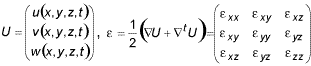The Hooke law is expressed by: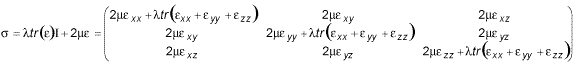With considering the harmonic wave exp(iωt), the displacement vector at an arbitrary point can be written as: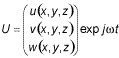Assuming plain strain, a cross-section of a plate is divided in the thickness direction into layered elements, and waves in the propagating direction z are described by the orthogonal function exp(iξz) where ξ is the wavenumber of the wave mode. The mth eigenvalue ξm of the eigensystem derived here denotes the wavenumber of the mth resonance mode. In the case of a bar with an arbitrary cross-section, the two dimensional cross-section is sub-discritized and waves in the longitudinal direction z are described by the orthogonal function exp(iξz).

The displacement vector is then expressed as: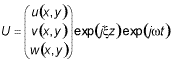Then the time dependence will be omitted as for the linear problem the time function is simplified: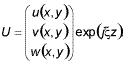Finally the virtual work principle gives the following governing equations: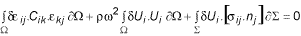In the appendix A the numerical details of the descritization and assembling of the virtual work is shown.

Kie and M are the assembled matrix over the geometry. The previous equation will be presented on the following form: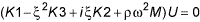An additional equation is then introduced: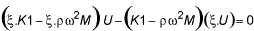The system of two equations is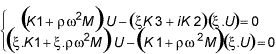The system matrix is finally expressed as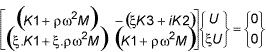Finally the virtual work equation is: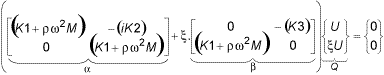Finally the virtual work equation is:The Eigen-values of the previous system correspond to the wave-numbers of the guided wave modes satisfying the resonant condition of this bar.

### 2.2 Implementation

The implementation of the previous formulation is developed in Matlab® platform based on sparse technique matrix.

The numerical program is using triangular finite elements and the Eigen-values are calculated based on numerical algorithm. The previous algorithm needs a starting solution for an Eigen-value calculation.

Some solutions are obtained negative or complex. The negative wave-numbers correspond to the propagation in the opposite direction. The complex solutions are evanescent and will be cancelled.

The scan of the surface (f, ξ) is obtained in three different ways:

• Calculate all the ξ for a fixed frequency by changing the starting solution.
• Calculate all the ξ for a fixed starting solution by changing the frequency.
• Using the previous solution ξ as a starting solution in the next step by changing the frequency.

When more than one ξ solution exists in a small frequency interval, some difficulties are observed in finding all of them. In this case a first scan type is more suitable. When no clear idea is available about the existing modes, the second scan type is used to find at least one mode per mode curve.

When aiming to calculate only one mode curve in the frequency range, the third scanning way is applicable and allows following the mode curve by using the present starting solution as the previous obtained. This calculation method presents further difficulties when the solution is NaN (not-a-number), in this case the next solution can not be obtained and the program is stopped. A combination of different ways is needed to find all the existing mode curves.

## 3. Validation

The validation of the numerical implementations is based on comparison with results from Disperse® solution in the case of circular rods (this validation is not shown in this paper).

### 3.1 Meshing criteria and validation

Good agreement has been also observed for the square rod dispersion curve calculation presented in Figure 1 and compared to the solution proposed by Takahiro in. Figure 1b shows good agreement up to 2.5 MHz*mm. The square dimension is 0.1*0.1m and we use the meshing of Figure 1a with 5 elements per square edge. The element length is 0.02m. For the non dimensional frequency/velocity validity range ([0..2.5 MHz mm], [0..5000m/s]) the minimum mode velocity is Cph=2900m/s, the minimum wavelength is about 0.011m. If we consider the mode Cph=4500m/s at the same frequency, the maximum wavelength is about 0.018m.

Clearly the mesh element length can be used as half the wavelength i.e L_elem=2λ, because the deformation of the section occurs along the axial direction. In the cross section the deformation remains easy to interpolate and so the meshing criteria is low compared to habitually used in transient simulation (L_elem<5λ).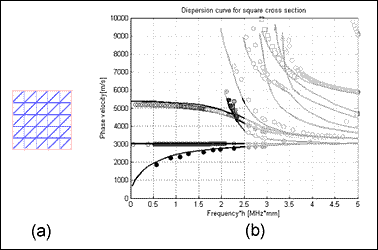Fig.1. Meshing and validity range of the meshing criteria for a steel square rod 3.2 I-Beam, numerical DC

The following example is a validation of the dispersion curve modelling in a complex cross section structure. The SAFEM method is used to deal with steel I-beam. This structure is generally used in many construction applications as reinforcement.

The Figure 2 shows the numerical modelling of the dispersion curves generated using the SAFEM, Figure 2b shows a complex number of dispersion curves in the range non dimensional frequency range[6,13] mHz.mm which corresponds to the frequency range of [40 kHz, 80 kHz]. This choice has been selected based on the bandwidth capability of the excitation transducer that will be used.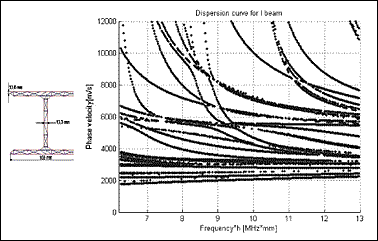Fig.2. Meshing and dispersion curves for steel I-beam in the frequency range [40 kHz, 80 kHz]

### 3.3 I-Beam, Experimental DC

The experimental dispersion curves were obtained for the I-beam (see Figure 3). A magnetostrictive (Ms) source was mounted at one end of the structure for excitation. The principle of the Ms source is based on the Ms effect which consists of a piece of ferromagnetic material which shrinks or elongates when a magnetic field is applied. For the experiments, some Ms strips were displayed on each side of the I-beam, then a coil made of copper was bonded to the structure. The coil, excited with a tone burst signal, produces an alternating magnetic field which expands and contracts the material underneath via the Ms effect and this generating the guided waves in the structure. For reception, a Micro Fibre Composite (MFC) sensor was set at the upper surface of the I-beam. Waveforms were recorded at 90 locations from L=240mm to L=1860mm, using 20 mm increment, where L represents the distance between the Ms source excitation and the MFC receiver (Figure 3).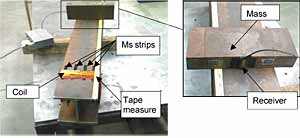Fig.3. Experimental setup of the source and receiver used in the I-beam inspection

The A-scans obtained for the different positions shows clearly the existence of a principal non dispersive mode travelling at the velocity 5225m/s, this mode is the less dispersive and the corresponding wave number is around 72 for the frequency of 60 kHz. Different modes are also shown in the Figure 4, they are superposed and the identification is very difficult to be achieved from the A-scan of the Figure 4. For this reason we use the advanced 2DFFT post processing aiming the identification and the separation of the different type of modes. The 2DFFT processing algorithm is described in .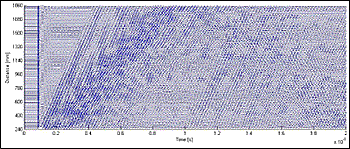Fig.4. Time distance A-scans acquisitions

Figure 5 presents the experimental result using the 2DFFT post processing, superposed to the SAFEM solution. Notice that the number of identified modes in the experimental results is less than the number achieved by the SAFEM, this is due to the excitation/reception transducer in use. In fact the MFC is only sensitive to a longitudinal displacement, and lose any information of displacement in the orthogonal direction, this means that any flexural mode is highly attenuated and should not appears in the DC plot.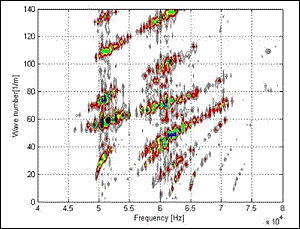Fig.5. Superposition of Experimental for steel I-beam obtained using 2DFFT / Numerical results of the same structure obtained using SAFEM

The main modes which appear to be less dispersive are very well represented in both experimental and numerical results, and the highest agreement is obtained for those modes. Those modes should have a high longitudinal displacement component and well detected by the MFC in use. For the most dispersive modes, the resolution of the time/position acquisitions should be higher to increase the accuracy of the experimental results.

## 4. Conclusion

In this work, we presented the SAFEM implementation for dispersion curves calculation of complex cross sections. The implementation is only performed for dispersion curves generation; the mode shape is then generated using the finite element software. The validation of the SAFEM was achieved with regards to published and also to experimental results.

This program has the benefit of the FEM capability to deal easily with the meshing of complex sections, in addition to the Matlab® solver capability in numerical nonlinear equation solution.

Validation of the program has been achieved with regard to experimental DC generation based on 2DFFT algorithm. Good agreement has been obtained in the frequency bandwidth allowed by the Ms source transducer.

## 5. Appendix

In the reference triangle finite element e the displacement is described as: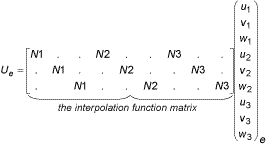Where N1 = 1 - ξ - η; N2 = ξ; N3 = η represent the linear interpolation functions.

The elementary strain description is obtained by the gradient operator applied to the displacement vector: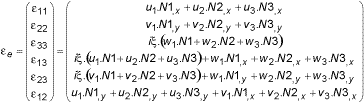then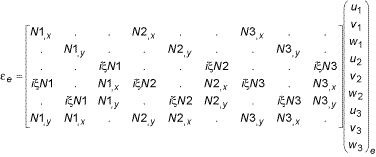(,d) represents the space derivative with respect to the direction (d). The inertia elementary term of (1) is: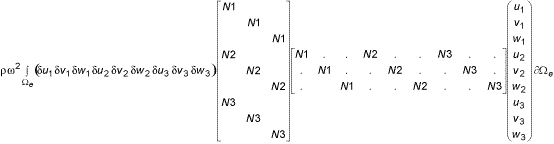The potential elementary term of the virtual work is: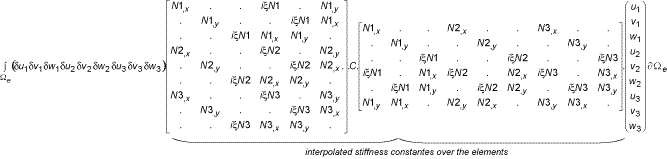Where C is given by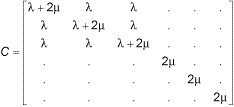The interpolated stiffness matrix over an element is expressed as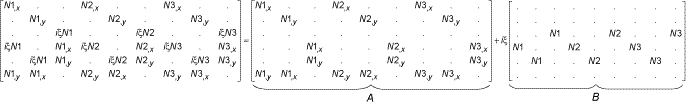The potential elementary term is expressed as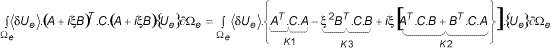In the absence of external forces, the virtual work is reduced to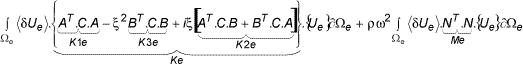### References

1. N.J. Nigro, Steady-state wave propagation in infinite bars of noncircular cross section, J. Acoust. Soc. Am. 40 (6) (1966) 1501- 1508.
2. V.V. Volovoi, D.H. Hodges, V.L. Berdichevsky, and V.G. Sutyrin, 'Dynamic Dispersion Curves for Non-Homogenous, Anisotropic Beams with Cross-Sectionsof Arbitrary Geometry,' Journal of Sound and Vibration 115, no. 5 (1998): 1101-1120.
3. L. Gavric, Computation of propagative waves in free rail using a finite element technique, J. Sound Vibrat. 187 (3) (1995) 531-543.
4. H. Taweel, S.B. Dong, M. Kazic, Wave reflection from the free end of a cylinder with an arbitrary cross-section, Int. J. Solids Struct. 37 (2000) 1701-1726.
5. T. Hayashi, K. Kawashima, Multiple reflections of Lamb waves at a delamination, Ultrasonics 40 (2002) 193-197.
6. T. Hayashi, J L Rose, guided wave simulation and visualization by a semi analytical finite element method. Material Evaluation, Jan (2003).
7. T. Hayashi, Won-Joon Song, Joseph L. Rose, Guided wave dispersion curves for a bar with an arbitrary cross-section, a rod and rail example, Ultrasonics 41 (2003) 175-183.
8. T. Hayashi, Chiga Tamayama, Morimasa Murase, Wave structure analysis of guided waves in a bar with an arbitrary cross-section, Ultrasonics 44 (2006) 17-24.
9. Yago GÓMEZ-ULLATE, Francisco MONTERO DE ESPINOSA, Selective Excitation of Lamb Wave Modes in Thin Aluminium Plates using Bonded Piezoceramics: Fem Modelling and Measurements, ECNDT (2006) - Poster 205.
10. D. Alleyne, P. Caeley, A two-dimensional Fourier transform method for the measurement of propagating signals. J. Acoust. Soc. Am. 89 (3), March (1991).
11. P. Moilanen, P. H. F. Nicholson, V. kilappa, S. Cheng, and J. Timonen, Measuring guided waves in long bones: modelling and experiments in free and immersed plates, Ultrasound in Med. & Biol., Vol. 32, No. 5, pp. 709-719, (2006).
12. R. M. Sanderson, Finite element analysis of guided waves in pipes and rails with flaws. Report number 13183.01/02/1154.03 TWI © MAY (2003).